# 效果预览：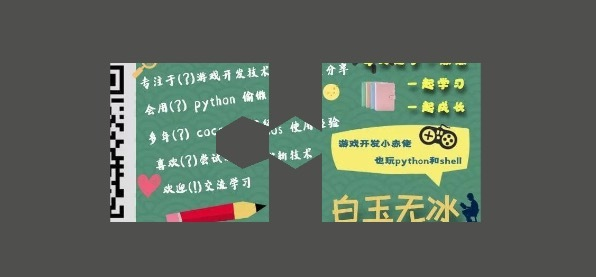# 使用方法：

1. 创建一个空节点
2. 添加用户脚本组件 `mesh-texture-mask`
3. 添加图片
4. 添加修改多边形顶点坐标

# 实现原理

## 创建 `mesh`

`mesh` 是什么？ `mesh` 是决定一个物体形状的东西。 例如在二维中可以是正方形、圆形、三角形等；在三维中可以是正方体、球体、圆柱体等。

`mesh` 初始化需要一个 `VertexFormat` 对象。这个对象是顶点格式对象。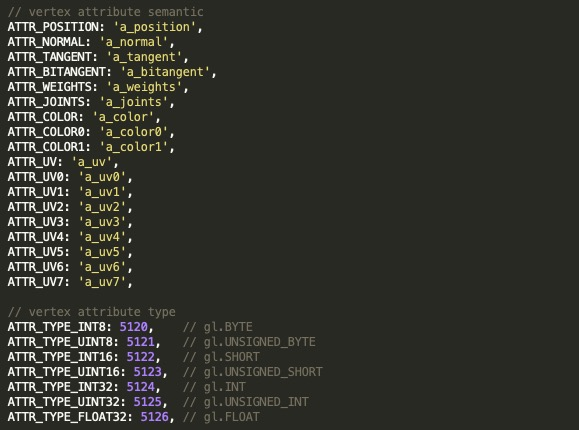`num` 对应有几个数据分量(猜的哈哈！)。例如二维坐标和纹理uv坐标一般只有`x``y`两个分量，所以设置为2；三维坐标有`xyz`三个变量，所以值为3；而颜色一般有 `rgba` 四个分量，所以设置为4。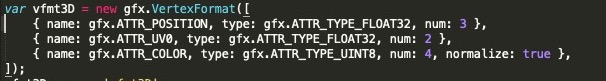`normalize` 表示归一化。

``````const gfx = cc.gfx;
let mesh = new cc.Mesh();
mesh.init(new gfx.VertexFormat([
{ name: gfx.ATTR_POSITION, type: gfx.ATTR_TYPE_FLOAT32, num: 2 },
{ name: gfx.ATTR_UV0, type: gfx.ATTR_TYPE_FLOAT32, num: 2 },
]), this.vertexes.length, true);
``````

## 计算纹理uv坐标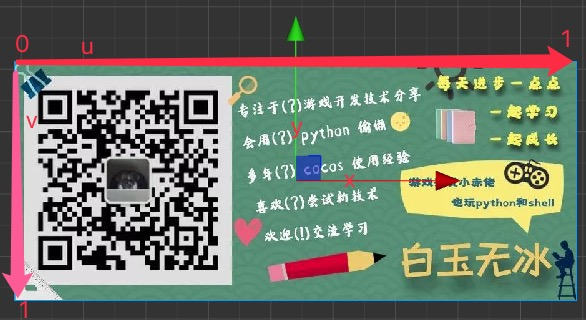``````const vx = (pt.x + this.texture.width / 2 + this.offset.x) / this.texture.width;
const vy = 1.0 - (pt.y + this.texture.height / 2 + this.offset.y) / this.texture.height;
``````

## 计算顶点索引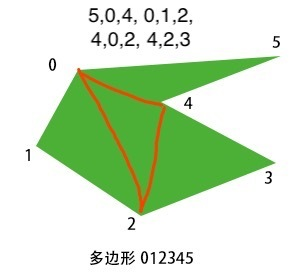``````const v1 = p2.sub(p1);
const v2 = p3.sub(p2);
if (v1.cross(v2) >= 0) {
// 是凸点
}
``````

``````// 判断一个点是否在三角形内
_testInTriangle(point, triA, triB, triC) {
let AB = triB.sub(triA), AC = triC.sub(triA), BC = triC.sub(triB), AD = point.sub(triA), BD = point.sub(triB);
return (AB.cross(AC) >= 0 ^ AB.cross(AD) < 0)  // D,C 在AB同同方向
&& (AB.cross(AC) >= 0 ^ AC.cross(AD) >= 0) // D,B 在AC同同方向
&& (BC.cross(AB) > 0 ^ BC.cross(BD) >= 0); // D,A 在BC同同方向
},
``````

# 小结

31赞

mark要确保顶点按顺序，边不相交。

2赞

M A R K

mark

mark

mark

mark

mark一下。学习